# Logik game

Letter game Logik is a two player game, which has the following rules:
1. The first player thinks five-letter word in which no letter is not
repeated.
2. The second player writes a five-letter word.
3. The first player answers two numbers - the first number indicates how many letters written word coincides with the imaginary word, and is also on the correct position; the second number indicates how many letters written word is contained in the imaginary word, but is not the right place.
4. Steps 2 and 3 are repeated until the second player imaginary word guess.
Recording one game of two friends looked like this:
SONET 1 2
MUDRC 0 2
PLAST 0 2
KMOTR 0 4
ATOLY 1 1
DOGMA 0 2

The next move was meant by the word guessed. Determine which word
it was.

Note:
Our presented solution contains a program in PHP language, which will solve the given combination of letters - by brute force, quickly and painlessly.

Result

x = (Correct answer is: STROM)### Step-by-step explanation:

x = STROM
```function match(\$s1, \$s2, \$q, \$w)
{
\$a = \$b = 0;
for(\$i=0;\$i<5;\$i++)
{
if(\$s1{\$i} == \$s2{\$i})
{
\$a++;
}
else
{
\$b += strpos(\$s2, \$s1{\$i})!==false;
}
}

return \$a == \$q && \$b == \$w;
}

for(\$a=65; \$a<91; \$a++)
{
for(\$b=65; \$b<91; \$b++)
{
for(\$c=65; \$c<91; \$c++)
{
for(\$d=65; \$d<91; \$d++)
{
for(\$e=65; \$e<91; \$e++)
{
\$s=chr(\$a).chr(\$b).chr(\$c).chr(\$d).chr(\$e);
if(!match(\$s,"SONET", 1, 2)) continue;
if(!match(\$s,"MUDRC", 0, 2)) continue;
if(!match(\$s,"PLAST", 0, 2)) continue;
if(!match(\$s,"KMOTR", 0, 4)) continue;
if(!match(\$s,"ATOLY", 1, 1)) continue;
if(!match(\$s,"DOGMA", 0, 2)) continue;

echo \$s;
exit;
}
}
}

}
}
```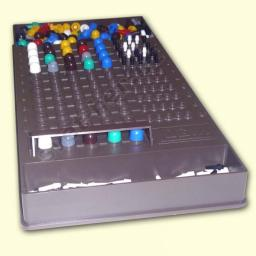Did you find an error or inaccuracy? Feel free to write us. Thank you!Tips to related online calculators
Would you like to compute count of combinations?

## Related math problems and questions:

• Five letters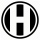How many ways can five letters be arranged?
• Phone numbersHow many 7-digit telephone numbers can be compiled from the digits 0,1,2,..,8,9 that no digit is repeated?
• Possible combinations - word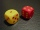How many ways can the letters F, A, I, R be arranged?
• PermutationsHow many 4-digit numbers can be composed of numbers 1,2,3,4,5,6,7 if: and the digits must not be repeated in the number b, the number should be divisible by five, and the numbers must not be repeated c, digits can be repeated
• The testThe test contains four questions, and there are five different answers to each of them, of which only one is correct, the others are incorrect. What is the probability that a student who does not know the answer to any question will guess the right answer
• Hazard game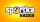In the Sportka hazard game, 6 numbers out of 49 are drawn. What is the probability that we will win: a) second prize (we guess 5 numbers correctly) b) the third prize (we guess 4 numbers correctly)?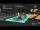The voltage station is day changing the master password, which consists of three letters. The code generation process does not change and is based on the following procedure: The following letters (A) to (I) correspond to different numbers from 1 to 9 if
• DigitsHow many natural numbers greater than 4000 which are formed from the numbers 0,1,3,7,9 with the figures not repeated, B) How many will the number of natural numbers less than 4000 and the numbers can be repeated?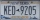A license plate has 3 letters followed by 4 numbers. Repeats are not allowed for the letters, but they are for the numbers. If they are issued at random, what is the probability that the 3 letters are in alphabetical order and the 3 numbers are consecutiv
• Big numbersHow many natural numbers less than 10 to the sixth can be written in numbers: a) 9.8.7 b) 9.8.0
• Four-digit numbersHow many four-digit numbers can we make from the numbers 2, 6, 3, 5, 1 and 9 if the numbers in the number cannot be repeated?
• How many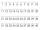How many double-digit numbers greater than 30 we can create from digits 0, 1, 2, 3, 4, 5? Numbers cannot be repeated in a two-digit number.
• WordsHow many 3 letter "words" are possible using 14 letters of the alphabet? a) n - without repetition b) m - with repetition
• STRESSED wordEach letter in STRESSED is printed on identical cards, one letter per card, and assembled in random order. Calculate the probability that the cards spell DESSERTS when assembled.
• DigitsHow many five-digit numbers can be written from numbers 0.3,4, 5, 7 that is divided by 10, and if digits can be repeated?
• Word MATEMATIKAHow many words can be created from the phrase MATEMATIKA by changing the letters' order, regardless of whether the words are meaningful?
• Hockey game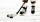In the hockey game was made six goals. Czech played against Finland. Czechs won 4:2. In what order to fall goals? How many game sequences were possible during the game?